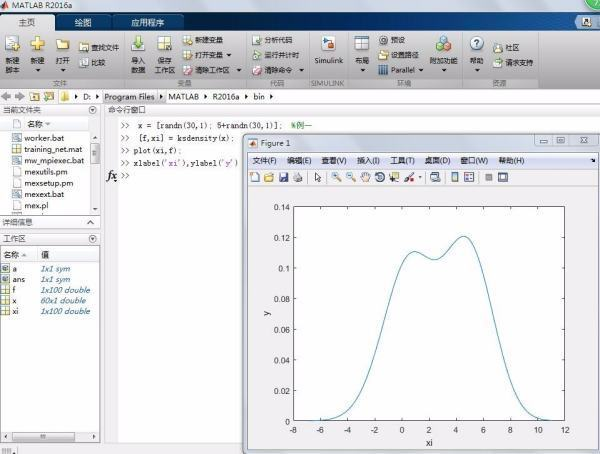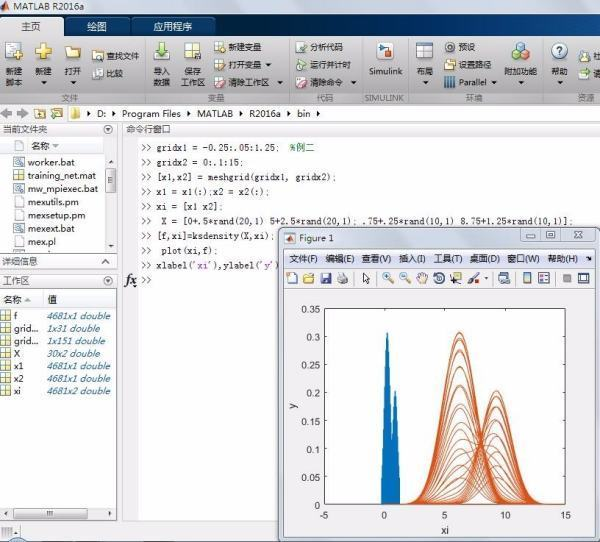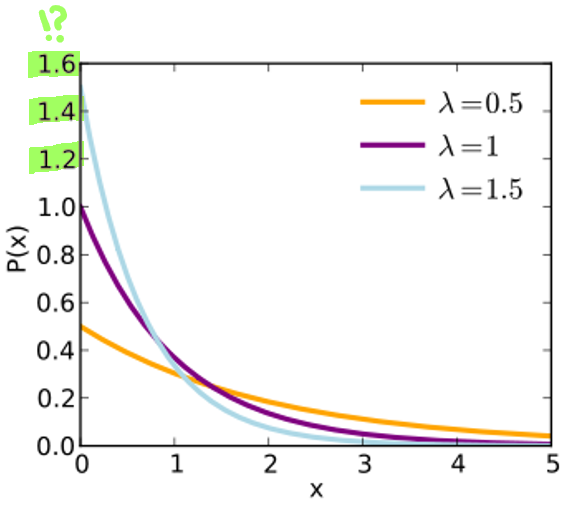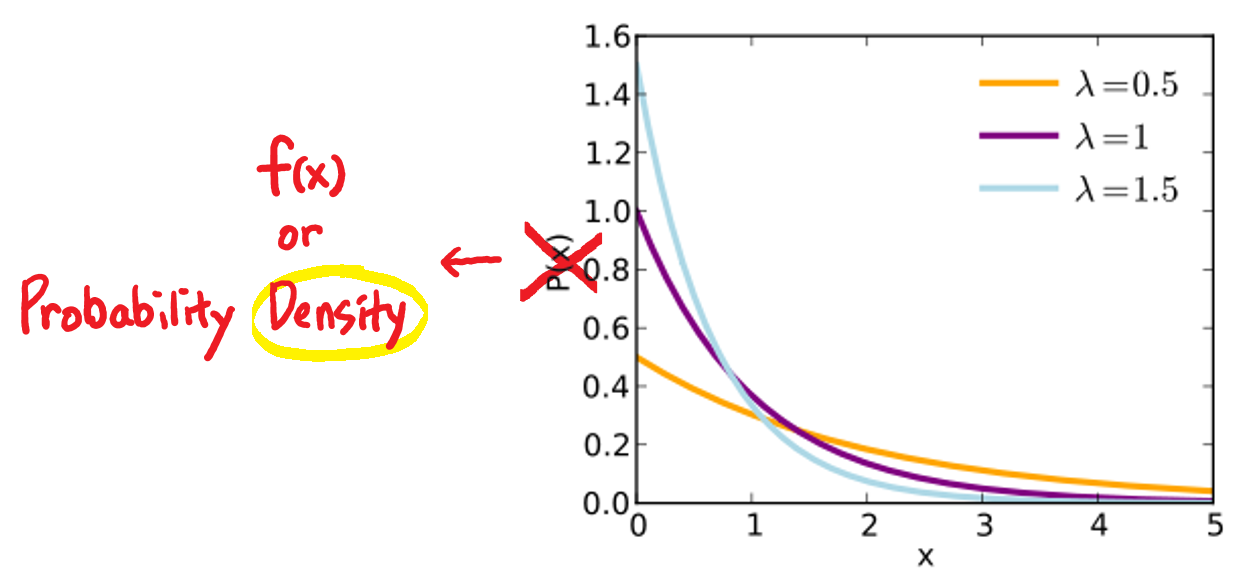• ksdensity函数用于计算一维或二维核密度或分布估计。其主要使用格式：[F,XI]=ksdensity(X) %计算的概率密度估计在向量或两列的矩阵X (ksdensity样本)评价100点密度估计(或二元数据的900点密度估计)的数据。式中：F...

ksdensity函数用于计算一维或二维核密度或分布估计。其主要使用格式：

[F,XI]=ksdensity(X)  %计算的概率密度估计在向量或两列的矩阵X (ksdensity样本)评价

100点密度估计(或二元数据的900点密度估计)的数据。

式中：F——密度值的向量。；XI——100(或900)点的集合。

例1：

x = [randn(30,1); 5+randn(30,1)];

[f,xi] = ksdensity(x);

plot(xi,f);

xlabel('xi'),ylabel('y')例2：

gridx1 = -0.25:.05:1.25;

gridx2 = 0:.1:15;

[x1,x2] = meshgrid(gridx1, gridx2);

x1 = x1(:); x2 = x2(:);

xi = [x1 x2];

X = [0+.5*rand(20,1) 5+2.5*rand(20,1);   .75+.25*rand(10,1) 8.75+1.25*rand(10,1)];

[f,xi]=ksdensity(X,xi);

plot(xi,f);

xlabel('xi'),ylabel('y')展开全文• 概率密度函数不是概率，它求和会大于1，甚至于单个的值也会大于1。如：x=randn(20)/100;[f,xi]=ksdensity(x(:));>> [xi' f']ans =-0.04043 0.0034258-0.039605 0.0071308-0.038779 0.013911-0.037954 0.025438...

概率密度函数不是概率，它求和会大于1，甚至于单个的值也会大于1。

如：

x=randn(20)/100;

[f,xi]=ksdensity(x(:));

>> [xi' f']

ans =

-0.04043    0.0034258

-0.039605    0.0071308

-0.038779     0.013911

-0.037954     0.025438

-0.037128     0.043652

-0.036303     0.070247

-0.035477      0.10615

-0.034652      0.15081

-0.033826      0.20215

-0.033001      0.25675

-0.032175      0.31059

-0.03135      0.36215

-0.030524      0.41313

-0.029699      0.47017

-0.028873      0.54456

-0.028048      0.65115

-0.027222      0.80361

-0.026397       1.0143

-0.025571         1.29

-0.024746       1.6339

-0.02392       2.0463

-0.023095       2.5261

-0.022269       3.0765

-0.021444       3.7071

-0.020618       4.4352

-0.019793       5.2807

-0.018967       6.2648

-0.018142       7.3979

-0.017316       8.6779

-0.016491       10.089

-0.015665       11.606

-0.01484       13.202

-0.014014       14.853

-0.013189       16.544

-0.012363       18.262

-0.011538       19.998

-0.010712       21.738

-0.0098869       23.459

-0.0090614       25.129

-0.0082359       26.715

-0.0074104       28.181

-0.0065849       29.501

-0.0057594        30.66

-0.0049339       31.661

-0.0041084       32.522

-0.0032829       33.271

-0.0024574        33.94

-0.0016319       34.553

-0.00080643       35.129

1.9064e-005       35.669

0.00084456       36.164

0.0016701       36.592

0.0024956       36.918

0.003321       37.097

0.0041465        37.08

0.004972       36.816

0.0057975       36.264

0.006623       35.395

0.0074485       34.198

0.008274       32.683

0.0090995       30.873

0.009925       28.811

0.010751        26.55

0.011576       24.156

0.012401       21.705

0.013227       19.279

0.014052       16.954

0.014878       14.798

0.015703       12.853

0.016529       11.139

0.017354       9.6479

0.01818       8.3543

0.019005        7.225

0.019831       6.2278

0.020656       5.3389

0.021482        4.545

0.022307       3.8415

0.023133       3.2286

0.023958        2.708

0.024784       2.2786

0.025609       1.9349

0.026435       1.6688

0.02726       1.4664

0.028086       1.3119

0.028911        1.187

0.029737       1.0744

0.030562      0.96175

0.031388      0.84102

0.032213      0.71198

0.033039      0.57921

0.033864      0.45042

0.03469      0.33352

0.035515      0.23432

0.036341      0.15592

0.037166     0.098122

0.037992     0.058277

0.038817     0.032698

0.039643     0.017284

0.040468    0.0086013

0.041294    0.0039524

>>

plot(f)

展开全文• 为什么MATLAB中的内核平滑函数ksdensity会导致值大于1？(Why Kernel smoothing function, ksdensity, in MATLAB, results in values greater than one?)我有一组样本，S，我想找到它的PDF。 问题是当我使用ksdensity...

为什么MATLAB中的内核平滑函数ksdensity会导致值大于1？(Why Kernel smoothing function, ksdensity, in MATLAB, results in values greater than one?)

我有一组样本，S，我想找到它的PDF。 问题是当我使用ksdensity时，我得到的值大于1！

[f,xi] = ksdensity(S)

在数组f中，大多数值都大于1！ 你能告诉我问题是什么吗？ 谢谢你的帮助。

例如：

S=normrnd(0.3035, 0.0314,1,1000);

ksdensity(S)

I have a set of samples, S, and I want to find its PDF. The problem is when I use ksdensity I get values greater than one!

[f,xi] = ksdensity(S)

In array f, most of the values are greater than one! Would you please tell me what the problem can be? Thanks for your help.

For example:

S=normrnd(0.3035, 0.0314,1,1000);

ksdensity(S)

原文：https://stackoverflow.com/questions/19086124

2019-11-30 09:11

满意答案

ksdensity ， ksdensity估计连续变量的概率密度函数。 概率密度可以大于1，它们实际上可以从零开始具有任意值。 对概率的约束是它们在可能的穷举范围内的总和必须是1.对于概率密度，约束是整个值范围内的积分是1。

可以在Matlab中获得由ksdensity估计的pdf的积分的粗略近似，如下所示：

sum(f) * min(diff(xi))

假设xi中的值是等间隔的。 该表达式的值应约为1。

如果在您的应用程序中您认为此近似值不足以接近1，则可能需要指定估计点网格(第二个参数pts )，使得间距更精细或范围比ksdensity自动生成的范围更宽。

ksdensity, as the name says, estimates a probability density function over a continuous variable. Probability densities can be larger than 1, they can actually have arbitrary values from zero upwards. The constraint on probabilities is that their sum over an exhaustive range of possibilities has to be 1. For probability densities, the constraint is that the integral over the whole range of values is 1.

A crude approximation of an integral of the pdf estimated by ksdensity can be obtained in Matlab like this:

sum(f) * min(diff(xi))

assuming that the values in xi are equally spaced. The value of this expression should be approximately 1.

If in your application you believe this approximation is not close enough to 1, you might want to specify the grid of estimation points (second parameter pts) such that the spacing is finer or the range is wider than the one automatically generated by ksdensity.

2013-09-30

相关问答

如果你“只需要一个x对平滑(y)”的阴谋，那么我建议在包stats考虑loess - 它很简单，快速和有效。 如果您真的想要基于内核平滑的回归，那么您可以在包np中的包KernSmooth或npreg中尝试locpoly 。 If you "simply want a plot of the x versus smoothed(y)", then I recommend considering loess in package stats - it's simple, fast and effec...

这是您的问题的明确解决方案： figure(1)

hold on; grid on

for i = 1:15

path = ['tmp_',num2str(i),'.out'];

fid = fopen(path, 'r');

a = textscan(fid,'%f');

% Appearantly the first line is a string in some of your files

% This if statement will take...

您可以传递一个匿名函数@(x,y) 1 - sum((x - y).^2 ./ (x + y) / 2) ./(x @(x,y) 1 - sum((x - y).^2 ./ (x + y) / 2)作为kernel_function参数。 You can pass an anonymous function @(x,y) 1 - sum((x - y).^2 ./ (x + y) / 2) as the kernel_function argument.

我认为这里的根本问题在于它不是需要达成一致的分布峰值，而是它们下面的区域。 此外，如果分布规范化，则该区域应等于1。 在上面的每个绘图方法中，“仓宽度”具有不同的尺寸，这导致每个仓中的值改变以便保留曲线下面积。 I think the fundamental thing here is that it's not the peaks of the distributions which need to agree, but the area under them. Also if the dist...

我建议使用类似的东西 d

library(mgcv)

m1

这将(1)尊重数据的二进制特性(2)使用广义交叉验证自动进行平滑度(术语中的“带宽”)选择。 使用 plot(y~x, data=d)

pp

pp\$y

我写了一个函数来解决我的问题。 这是我称之为kernelsmooth的功能。 它需要三个参数，一个要平滑的数据矩阵，一个内核和一个布尔标志范数 ，如果为真，则通过其值的平均值对内核进行标准化(防止矩阵数据膨胀)。 kernelsmooth

# how many rows/cols of zeroes are used to pad.

width

这更接近你的期望吗？ ksdensity函数需要来自分布的样本向量，而您正在为它提供概率密度函数的值。 >> xi = -3:0.1:3;

>> p1 = normpdf(xi, 1, 0.3);

>> p2 = normpdf(xi,-1, 0.3);

>> subplot(211)

>> plot(xi, 0.5*p1+0.5*p2)

>> a1 = 1 + 0.3 * randn(10000,1); % construct the same distribution

>> a2 = -1 ...

排除卷积中的坏点很容易。 如果M2包含您要包含的像素为1 ，而对于那些不包含的像素包含0 ，那么您只需执行以下操作： newImage = conv2(A.*M2, M1,'same');

这可能足以满足您的需要，但您必须确定“平均”的确切含义。例如，给定您的内核ones(10) ，最后您可能需要执行以下操作： npts = conv2(ones(size(A)).*M2, M1, 'same')

newImage = newImage./npts

即，将每个像素除以实际包含在该像素的卷积中的...

好的，我发现了这个问题。 我只需要将gaussfilter的FFT与gaussfilter相乘，然后将标志'replicate'传递给imfilter 。 变化： testmatrix1 = testmatrix1 .* fftshift(fftn(ifftshift(gaussfilter),[]));

和 testmatrix2 = imfilter(testmatrix, gaussfilter,'replicate');

Ok, I found the issue. I just ha...

ksdensity ， ksdensity估计连续变量的概率密度函数。 概率密度可以大于1，它们实际上可以从零开始具有任意值。 对概率的约束是它们在可能的穷举范围内的总和必须是1.对于概率密度，约束是整个值范围内的积分是1。 可以在Matlab中获得由ksdensity估计的pdf的积分的粗略近似，如下所示： sum(f) * min(diff(xi))

假设xi中的值是等间隔的。 该表达式的值应约为1。 如果在您的应用程序中您认为此近似值不足以接近1，则可能需要指定估计点网格(第二个参数pts...

相关文章

中文名: MATLAB及应用 作者: 胡鹤飞 图书分类: 软件 资源格式: PDF

...

http://helephant.com/2012/07/14/javascript-function

...

中文名: MATLAB智能算法30个案例分析 作者: 史峰 王辉 郁磊 胡斐

...

中文名: 模式识别与智能计算：MATLAB技术实现(第2版) 作者: 杨淑莹 图书分类:

...

中文名: 数字图像处理与机器视觉：Visual C++与Matlab实现 作者: 张铮 图

...

以下是代码： #include<iostream>using namespace std

...

作用： 作为 table 的子节点，用于声明路由规则 属性： ref – Function 类型的 b

...

Linux Kernel BUG:soft lockup CPU#1 stuck分析 1.线上内核bu

...

List的size大于0，但是取到的值为null，谁见过这种情况？ List list=XXDAO.

...

最新问答

这是你的答案： http://jsfiddle.net/gPsdk/40/ .preloader-container { position: absolute; top: 0px; right: 0px; bottom: 0px; left: 0px; background: #FFFFFF; z-index: 5; opacity: 1; -webkit-transition: all 500ms ease-out;

问题是，在启用Outlook库引用的情况下， olMailItem是一个保留常量，我认为当您将Dim olMailItem as Outlook.MailItem ，这不是问题，但是尝试设置变量会导致问题。 以下是完整的解释： 您已将olMailItem声明为对象变量。 在赋值语句的右侧，在将其值设置为对象的实例之前，您将引用此Object 。 这基本上是一个递归错误，因为你有对象试图自己分配自己。 还有另一个潜在的错误，如果之前已经分配了olMailItem ，这个语句会引发另一个错误(可能是

我建议使用wireshark http://www.wireshark.org/通过记录(“捕获”)设备可以看到的网络流量副本来“监听”网络上发生的对话。 当您开始捕获时，数据量似乎过大，但如果您能够发现任何看起来像您的SOAP消息的片段(应该很容易发现)，那么您可以通过右键单击并选择来快速过滤到该对话'关注TCP Stream'。 然后，您可以在弹出窗口中查看您编写的SOAP服务与Silverlight客户端之间的整个对话。 如果一切正常，请关闭弹出窗口。 作为一个额外的好处，wireshar

Android默认情况下不提供TextView的合理结果。 您可以使用以下库并实现适当的aligntment。 https://github.com/navabi/JustifiedTextView Android Does not provide Justified aligntment of TextView By default. You can use following library and achieve proper aligntment. https://github.com/

你的代码适合我： class apples { public static void main(String args[]) { System.out.println("Hello World!"); } } 我将它下载到c：\ temp \ apples.java。 以下是我编译和运行的方式： C:\temp>javac -cp . apples.java C:\temp>dir apples Volume in drive C is HP_PAV

12个十六进制数字(带前导0x)表示48位。 那是256 TB的虚拟地址空间。 在AMD64上阅读wiki(我假设你在上面，对吗？)架构http://en.wikipedia.org/wiki/X86-64 12 hex digits (with leading 0x) mean 48 bits. That is 256 TB of virtual address space. Read wiki on AMD64 (I assume that you are on it, right?) ar

这将取决于你想要的。 对象有两种属性：类属性和实例属性。 类属性 类属性对于类的每个实例都是相同的对象。 class MyClass: class_attribute = [] 这里已经为类定义了MyClass.class_attribute ，您可以使用它。 如果您创建MyClass实例，则每个实例都可以访问相同的class_attribute 。 实例属性 instance属性仅在创建实例时可用，并且对于类的每个实例都是唯一的。 您只能在实例上使用它们。 在方法__init__中定

展开全文• 概率密度函数（PDF)不是概率 probability density function v.s. probability 概率密度函数在x点的取值可能大于1，那么它是如何积分和取到1？ 已知一个点最大的概率是1，但是对于一些概率密度函数中，比如指数分布的...

https://towardsdatascience.com/pdf-is-not-a-probability-5a4b8a5d9531

概率密度函数（PDF)不是概率

probability density function v.s. probability

概率密度函数在x点的取值可能大于1，那么它是如何积分和取到1？

已知一个点最大的概率是1，但是对于一些概率密度函数中，比如指数分布的PDF中，PDF能取到1.5如果PDF函数的对应x的取值并非在负无穷和正无穷取满，而是只取一小段，其他x对应的PDF为0，那么是可以积分和为1的。如下图，均匀分布，在【0,0.5】之间取值PDF=2，其余为0，则成立。

PDF 和 probability 之间的区别

首先搞清楚probability mass function 的概念： 离散随机变量取某个值时的概率函数。
PMF PDF的区别在于后者是连续的而非离散的。
离散随机变量和连续随机变量的概率不是同样定义的
对于离散随机变量，我们认为某个值的PMF是概率。
对于连续随机变量，我们取一段区间的PDF积分作为X落在这个区间的概率。需要注意，对于连续随机变量，X等于某一个具体值的概率为0，

为什么对于连续随机变量，X等于某一个具体值的概率为0，而PDF不为0？

The probability density at x=1 is two.
But why is the probability at x=1 zero?

有一种通俗的方法去理解为何连续随机变量上的点概率为0。因为任意小的区间也可以由无穷个点构成，故每个点的概率可以近乎于0，但这种解释不可以做为正式解释。
只能说，
there are different definitions of probabiltiy in discrete and continous variables.
For discrete random variables, the probabiltiy is defined as the discrete PMF at point, that is the probability mass function that x equals to a certain point, and it 's less than 1.
For continous random variables, the probability is defined as the integral PDF of interval, that is the probability density function that x falls into a certain interval, and it can be greater than1.

对于连续随机变量，我们如何由PDF计算probability?

我们已知，对于连续随机变量，任一点的概率是0，那么这一点的细微区间的概率可以作为这一点的概率。
P ( X = x ) = ∫ x x + d x f ( x ) d x P(X=x)=\int _{x}^{x+dx} f(x)dx
其中f(x)是这一点的概率密度函数PDF，而非这一点的概率。

那么PDF意味着什么？ Probability density at point x

它意味着x附近的概率的陡峭程度，而非那一点的概率。

PDF坐标轴的名称不可以是概率，而应该是概率密度展开全文• seid=16338053070486680597&...%%绘制直方图+概率密度函数+累积分布函数 %矩阵A是random生成0-1的随机数，或读入自己的数据 A = rand(1,500)*50; %求A的最大、最小、均值 maxA = max(A); minA = min(A); meanA = meanmatlab 开发语言 统计模型
• 核密度估计（kernel density estimation）是在概率论中用来估计未知的密度函数，属於非参数检验方法之一，由Rosenblatt (1955)和Emanuel Parzen(1962)提出，又名Parzen窗（Parzen window）。Ruppert和Cline...KDE
• %% %数据统计与分析 %产生随机变量，计算概率密度，计算概率分布，概率统计特征，有关概率的作图 %% %产生随机变量 n=10:10:60; R=binornd(n,1./n,1,6)%服从二项分布...%自定义函数概率密度计算ksdensity() clear;...